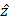# The region between y = 0 and y = d contains a magnetic field= B. A particle of mass m and charge q enters the region with a velocity= v. If d =, the  acceleration of the charged particle at the point of its emergence at the other side is  :- a.b.c.d. none of these

## Question ID - 50388 :- The region between y = 0 and y = d contains a magnetic field= B. A particle of mass m and charge q enters the region with a velocity= v. If d =, the  acceleration of the charged particle at the point of its emergence at the other side is  :- a.b.c.d. none of these

3537

None of these

Next Question :
 Astronauts in space can't stand at one place, because (a) there is no gravity (b) viscous forces of the atmosphere are very strong (c) solar wind exert an upward force (d) atmospheric pressure is very low